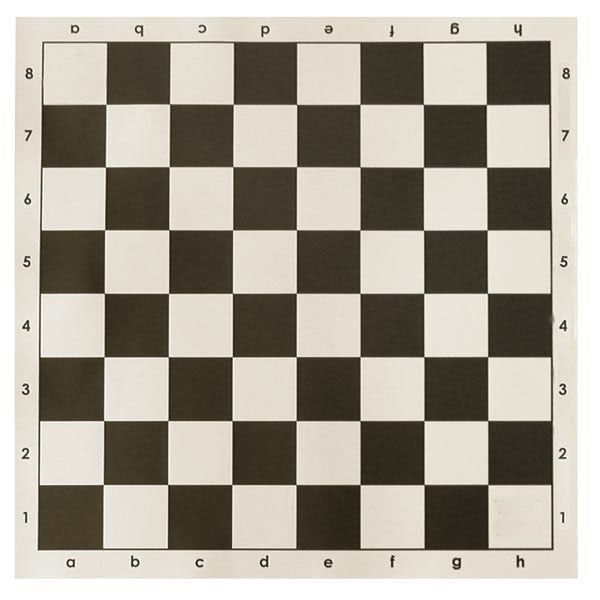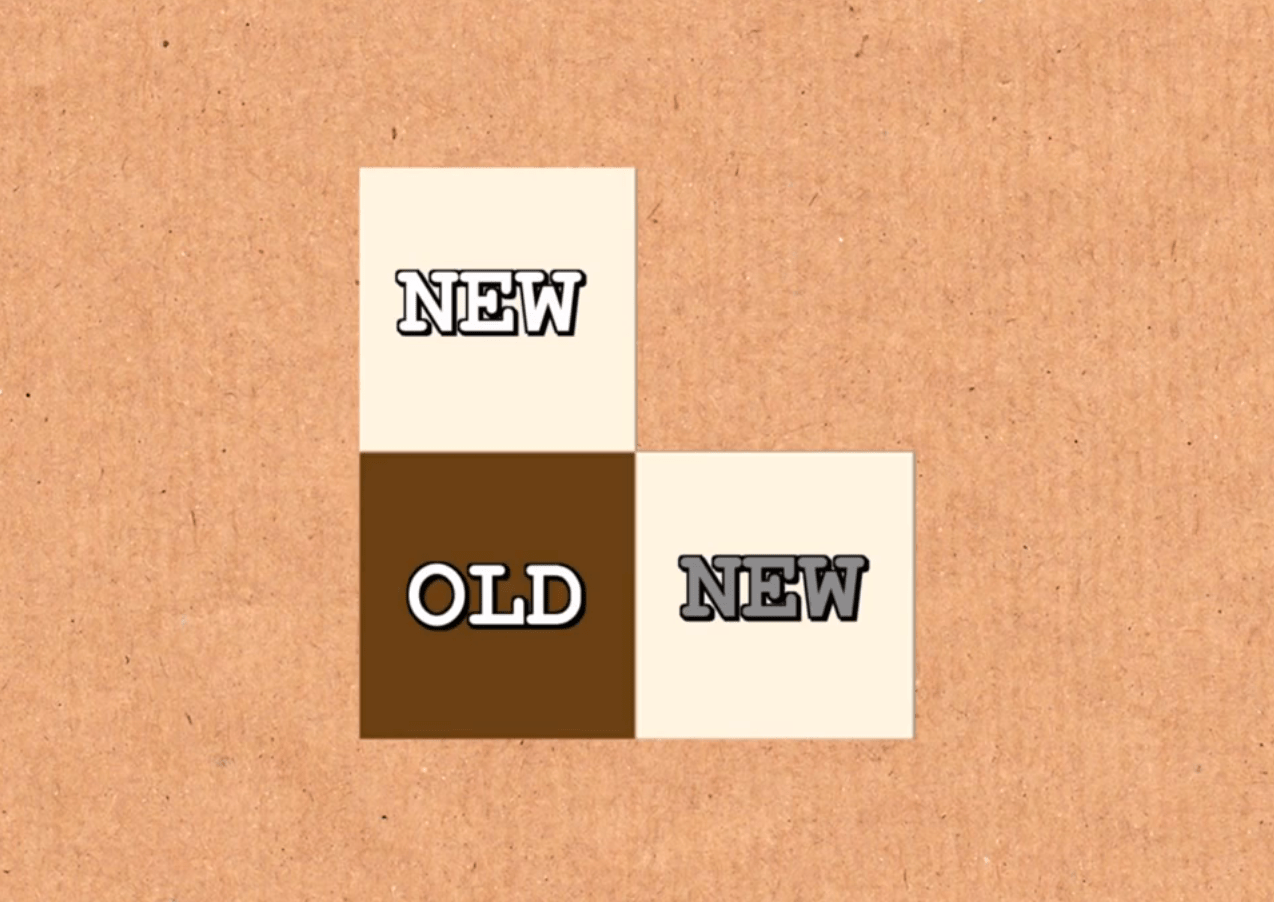# 棋盘上的越狱游戏.

.

.

.

.

.

.

.

.

.$$S=1+1/2+1/4+1/8+\dots$$

$$2S=2+1+1/2+1/4+1/8+\dots$$

$$2S=2+S$$

$$A=9/10+9/100+9/1000+9/10000+\dots$$$$9/10+9/100+9/1000+9/10000+\dots=0.9+0.09+0.009+0.0009+\dots=0.99999999999 \dots$$

$$0.99999999\dots=1$$

## 《棋盘上的越狱游戏》有1个想法

1.momo说道：

数学归纳法？